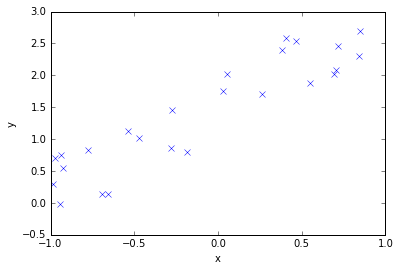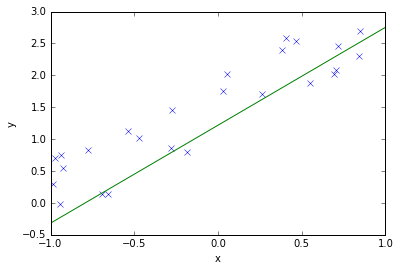## Maximum Likelihood Principle for deriving cost functions for regression and classification¶

### Hypothesis set¶

Given a set of hypotheses $\mathcal H$ (here parametric models) for explaining some data $\mathcal D$. The hypotheses in a set should have the same functional form, but have different parameters $\theta$.

As an example we assume that the data are $(x,y)$-pairs (like in supervised learning):

$\mathcal D = \{ (x_1, y_1), (x_2, y_2), \dots , (x_n,y_n) \}$

### Example: (Linear) Regression¶

Let's generate some data (xy-pairs):

In :
import numpy as np
x = np.random.rand(25) * 2.  - 1.
y = 1. + 1.5 * np.sin(x) + np.random.rand(len(x)) * 1.
%matplotlib inline
import matplotlib.pyplot as plt
plt.plot(x, y, 'bx')
plt.xlabel('x')
plt.ylabel('y')

Out:
<matplotlib.text.Text at 0x10ac6c6d0>Just looking at the graph, a linear relation seem to be a good fit. (In fact the noise is much stronger than the non-linearity of the sin in the given x-range.)

A plausible assumption for the noise of real world data is that the noise is gaussian $\mathcal N (0,\sigma$), because of the Central Limit Theorem. If there are many factors which influences the noise, each of them can be seen as a step of a one-dimesional random walk. The probability distribution of a one-dimesional random walk is a gaussian distribution (see for instance: Reif: Fundamentals of Statistical and Thermal Physics)

The hypothesis set $\mathcal H$ of a linear model:

$$h_\theta(x) = \theta_0 + \theta_1 x$$

So $\mathcal H$ is parametrized by $\theta = \{\theta_0, \theta_1 \}$.

The probability $p(y \mid x)$ is given by:

$$p(y \mid x) = h_\theta(x) + \mathcal N(0, \sigma^2)$$

This is a discriminative model $p(y \mid x)$. We have a probability for $y$ given $x$ and some fixed parameters. (The variance of the noise $\sigma^2$ can also be seen as a parameter of the model.)

## Bayes Theorem¶

Given a hypothesis set $\mathcal H$ and some data $\mathcal D$ the probability of the parameters $\theta$ can be expressed by the bayes theorem:

$$P(\theta \mid \mathcal D, \mathcal H) = \frac{P(\mathcal D \mid \theta, \mathcal H) \cdot P(\theta \mid \mathcal H)}{P(\mathcal D \mid \mathcal H)}$$

For this problem the different parts of the equation have the following names: $$posterior = \frac{likelihood \cdot prior}{evidence}$$

### Maximum Likelihood Estimator¶

A maximum likelihood estimator for the parameters considers only the likelihood $P(\mathcal D \mid \theta, \mathcal H)$ to get an estimation for the parameters $\theta$ by maximizing the likelihood.

$$\underset{\theta}{\operatorname{argmax}} P(\mathcal D \mid \theta, \mathcal H)$$

For a constant prior the following holds (follows from bayes rule):

$$\underset{\theta}{\operatorname{argmax}} P(\theta \mid \mathcal D, \mathcal H) = \underset{\theta}{\operatorname{argmax}} P(\mathcal D \mid \theta, \mathcal H)$$

Note: The denominator $P(\mathcal D\mid \mathcal H)$ is independent of $\theta$, so it plays no role in the maximization.

##### Example: Regression¶

For a discriminative model we use the conditional likelihood $P(Y \mid X, \theta, \mathcal H)$ (instead of $P(\mathcal D \mid \theta, \mathcal H)$)

For our regression example note that each data point is conditional independent from the others under the hypothesis set $\mathcal H$ (for a fixed $\theta$).

$$\underset{\theta}{\operatorname{argmax}} \left[ P(y_1\mid x_1; \theta, \mathcal H) \cdot P(y_2\mid x_2; \theta, \mathcal H) \dots \cdot P(y_n\mid x_n;\theta, \mathcal H) \right]$$ with

$$P(y_i\mid x_i; \theta, \mathcal H) = h_\theta(x_i) + \mathcal N(0, \sigma^2) = \mathcal N(h_\theta(x_i), \sigma^2) = \frac {1}{\sigma \sqrt{2\pi}} e^{- \frac{(y_i - h_\theta(x_i) )^2 }{2 \sigma^2}}$$

The maximizer of the likelihood is the same as the minimizer of the negative log-likehihood, because the logarithm is a monotonic function.

$$\underset{\theta}{\operatorname{argmin}} \left[- \log(P(y_1\mid x_1; \theta, \mathcal H)) - \log(P(y_2| x_2; \theta, \mathcal H)) - \dots - \log(P(y_n\mid x_n ;\theta, \mathcal H)) \right]$$

with $$\log(P(y_i \mid x_i; \theta, \mathcal H)) = \log(\mathcal N(h(x_i), \sigma)) = C -\frac{(y_i - h_\theta(x_i))^2}{2 \sigma ^2}$$

The argmin is independent on the constant $C$ and with the assumption that each data point has the same $\sigma^2$ the maximum likelihood estimation of the parameters $\theta$ is equivalent to the minimization of the squared error:

$$E = \underset{\theta}{\operatorname{argmin}} \frac{1}{2}\sum_i (y_i - h_\theta (x_i))^2$$

Note that we didn't used the linear relationship between $x$ and $y$. So that's valid for regression in general.

Therefore the MLE (maximum likelihood estimation) for finding the parameters of a parametric regression is equivalent to the minimization of the squared error.

This implies a gaussian noise assumption.

For example from numpy's polyfit documentation:

Fit a polynomial p(x) = p * x**deg + ... + p[deg] of degree deg
to points (x, y). Returns a vector of coefficients p that minimises
the squared error.
In :
theta_0, theta_1 = np.polyfit(x, y, deg=1)

x_ = np.array([-1.,1])
y_ = theta_0 + theta_1 * x_

plt.plot(x, y, 'bx')
plt.plot(x_,y_, 'g-')
plt.plot
plt.xlabel('x')
plt.ylabel('y')

Out:
<matplotlib.text.Text at 0x10adf3490>### Example: Binary Classification¶

For binary classification the train data $\mathcal D$ consists of $(\vec x^{(i)}, t^{(i)})$ pairs (with $t^{(i)} \in \{0,1\}$).

The hypothesis $h(\vec x^{(i)})$ gives us the probability that the feature vector $\vec x$ corresponds to an object of the positive class, i.e. $p$ for $y^{(i)}=1$:

$$p(y^{(i)}=1 \mid \vec x^{(i)}) = h(\vec x^{(i)})$$

and for the negative class

$$p(y^{(i)}=0 \mid \vec x^{(i)}) = 1 - p(y^{(i)}=1 \mid \vec x^{(i)}) = 1- h(\vec x^{(i)})$$

Each data point is conditional independent from the others under the hypothesis set $\mathcal H$ as for the regression example. So the maximum likelihood principle gives us:

$$\underset{\theta}{\operatorname{argmax}} P(\mathcal D| \theta, \mathcal H) = \underset{\theta}{\operatorname{argmax}} \prod_{i} \left(h(\vec x^{(i)})\right)^{t^{(i)}} \left(1-h(\vec x^{(i)})\right)^{1-t^{(i)}}$$

Note: The 'to the power' trick selects the right probability prediction $h$ resp. $1-h$.

So the corresponding minimization of the negative log likelihood is given by:

$$\underset{\theta}{\operatorname{argmin}} \left( - P(\mathcal D| \theta, \mathcal H) \right)= \underset{\theta}{\operatorname{argmin}} \left( - \sum_{i} \left( {t^{(i)}} \log (h(\vec x^{(i)}) + {(1-t^{(i)})} \log(1-h(\vec x^{(i)} ) \right)\right) $$

The cost function is also called cross entropy cost for binary classification (see below) So the maximum likelihood principle for binary classification is equivalent to minimizing the (binary) cross entropy.

#### What's exactly the relation to cross entropy?¶

Definition of the cross entropy $H(p,q)$ between two distributions $p(y \mid \vec x)$ and $q(y \mid \vec x)$:

$$H(p,q) := - \mathbb E_{(\vec x,y)\sim p}[\log q(y \mid \vec x)] = - \sum_y \int_{\mathcal X} p(y \mid \vec x) \log q(y \mid \vec x) d\vec x$$

with

• $\mathcal X$: $\vec x \in \mathbb R^n$
• $y \in \{0,1\}$

For a binary classification problem with one output $h(\vec x)$ of the classifier, the probability for the positive class $y=1$ is the output $h(\vec x)$:

• $q(y=1 \mid \vec x) = h(\vec x)$
• $q(y=0 \mid \vec x) = 1- h(\vec x)$
• $p(y\mid x)$: "true" unknown conditional probability

So the cross entropy becomes: $$H(p,q) = - \mathbb E_{(\vec x,y)\sim p}[\log q(y \mid \vec x)] = - \int_{\mathcal X} p(y=1 \mid \vec x) \log h(\vec x) d\vec x - \int_{\mathcal X} p(y=0 \mid \vec x) \log \left(1-h(\vec x)\right) d\vec x$$

Sampling of $m$ training examples $(\vec x^{(i)}, t^{(i)})$ from the distribution $p(\vec x,y)$ and labeling with the true label $t$ we can estimate the cross entropy $J \approx H(p,q)$ by:

$$J = - \frac{1}{m} \sum_{i=1}^m \left(\mathbb 1_{t^{(i)}=1}\log h(\vec x^{(i)}) + \mathbb 1_{t^{(i)}=0} \log \left(1-h(\vec x^{(i)})\right) \right)$$ this is equivalent to $$J = - \frac{1}{m} \sum_{i=1}^m \left(t^{(i)}\log h(\vec x^{(i)}) + \mathbb (1-t^{(i)}) \log \left(1-h(\vec x^{(i)})\right) \right)$$

So minimization of the negative log likelihood is equivalent to the minimization of the cross entropy.

Because of $$D_{KL}(p \mid \mid q) = H(p,q) - H(p)$$

with

• $D_{KL}$: Kullback Leibler Divergence
• $H(p)$: Entropy

Minimizing the cross entropy is equivalent to minimizing $D_{KL}$. For fixed $p$ minimizing $H(p,q)$ is equivalent to minimizing $D_{KL}$. The minimal possible $D_{KL}$ is $0$. That holds if the distributions $p$ and $q$ are the same, i.e. $p=q$.